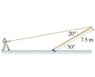# Torque Equilibruim Problem

• thousandsaints

#### thousandsaints

I really need help understanding this problem. I'd be really thankful if someone could explain it to me.

## Homework Statement

A man is attempting to raise a 7.5 m long, 29 kg flagpole that has a hinge at the base by pulling on a rope attached to the top of the pole. The figure of the problem is below as an attachment

With what force does the man have to pull on the rope to hold the pole motionless in this position?

## Homework Equations

$$\tau$$=F*r$$\bot$$

Net torque = 0

## The Attempt at a Solution

I tried finding the torque caused the weight of the flagpole, and then the torque caused by the man. From that I tried finding the force which the man exerts. But I can't find the correct answer.

Torque from gravity = w*r$$\bot$$ =mg*Lcos($$\Theta$$) =(9.81*29)*7.5cos30 = 1848 Nm

Torque from the man = F*r$$\bot$$ = F*Ltan$$\Theta$$ = F*7.5tan20 = F*2.73m

The two torque values should equal each other. so...

1848 Nm = F*2.73m
F= 1848 Nm / 2.73
F= 676.8 N

#### Attachments

•problem.jpg
4.7 KB · Views: 778
Last edited:
What is the question asking for? You haven't really stated that.

Your general method will work assuming your trying to find equilibrium (i.e. the pole isn't moving).
The problem with the details of your calculation however, is the gravity.
For any uniform (in density) object, you can treat the force of gravity as acting on the center of mass (not the end of the pole which you have done in the equation).

It might help if you draw a free body diagram of the pole, to help visualize the difference in radii.

sorry about that. I edited the post above.

The question is:
With what force does the man have to pull on the rope to hold the pole motionless in this position?

Welcome to PF!

Torque from gravity = w*r =mg*Lcos() =(9.81*29)*7.5cos30 = 1848 Nm

Torque from the man = F*r = F*Ltan = F*7.5tan20 = F*2.73m

Hi thousandsaints! Welcome to PF!(You haven't said, but I assume you're taking torques about the end of the pole on the ground).

In the first equation, you only need half the length (as lzkelley says)!In the second equation, I suspect you've drawn the wrong right-angled triangle … it isn't tan20.